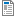National Curriculum

# Year 4 Maths Plans

We provide Hamilton Year 4 maths both as weekly plans (below) and as short blocks. We will eventually be phasing out the plans, as we believe our short blocks offer you all of the same advantages and more. Find out more about the advantages of Hamilton's short blocks.

Supporting documents for set
• Week
• Title
1
+ Details
Place value

Understand what each digit represents in a 4-digit number. Write place value additions and subtractions. Place 3-digit and 4-digit numbers on a line and compare them.Autumn Week 1 Plan
2
+ Details

Mental addition of pairs of 2-digit numbers or 3-digit numbers and 2-digit numbers using partitioning, place value or counting up. Mental subtraction of 2-digit numbers from numbers less than 200 by counting back or counting up. Includes bar model examples.Autumn Week 2 Plan
3
+ Details

Written addition of 3-digit numbers using compact written addition. Mental subtraction by counting up, using Frog and checking with addition. Includes bar model examples.

4
+ Details
Shape and data

Draw circles with different radii. Describe, name and sort 2D and 3D shapes, including triangles. Use Venn diagrams or Carroll diagrams to sort shapes.

5
+ Details
Multiplication and division

Mental multiplication and division: Double 2-digit and 3-digit numbers using partitioning. Halve 2-digit and 3-digit numbers using partitioning. Know 3, 4, 6, 8 times tables and associated division facts. Includes bar model examples.

6
+ Details

Mental addition and subtraction of 4-digit numbers using place value and partitioning. Add and subtract 1, 10, 100, 1000 to and from 4-digit numbers.

7
+ Details

Mental addition and subtraction of 3 or 4-digit numbers using place value and near multiples.

8
+ Details
Subtraction

Written subtraction of 3-digit numbers using expanded decomposition. Mental subtraction using counting up. Choose a subtraction calculation strategy.

9
+ Details
Time and data

Tell the time to the nearest minute; use analogue, digital and Roman numeral clocks. Use am and pm times appropriately. Convert units of time and work out time intervals crossing the hour. Collect data and organise into bar charts and pictograms.

10
+ Details
Multiplication and division

Written multiplication using grid method to multiply a 2-digit number by a 1-digit. Written division use chunking on a number line to divide with no remainders.

11
+ Details
Division and fractions

Written division of 2-digit numbers by 1-digit numbers, just above the 10th multiple with remainders using chunking. Count ins 1/4s, 1/3s, 1/8s and 1/10s saying the equivalent fractions, e.g. 1 ½ not 12/4. Find unit fractions and non-unit fraction of amounts. Includes bar model examples.